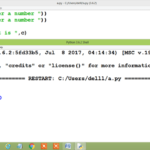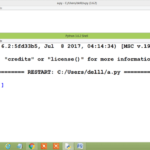# Python program to add two numbersIntroduction: In this article, I am going to explain a Python program to add two numbers. If you are trying to use python operators then the Python program to add two numbers is a good beginning. There are several types of operators available in python but if you want to learn python arithmetic operators then Python program to add two numbers is a good choice. Algorithm: Here, I am going to explain the logic of program with the help of a simple algorithm Here, I am going to explain ...

# How to take space separated input in Python?Introduction: In this article I am going to explain  how to take space separated input in Python?  There may be a situation when we want to enter more than one values but all the  values should be separated by space and we hit the enter key after the last input.  To fulfill this requirement we should know that how to take space separated input in Python.  We are using several types of input devices for user input .  The standard input device is a keyboard.  In t...

# Python Program to Check Whether a Number is Prime or NotIn this article, we will check whether an integer is a prime no or not in python. What is Prime Number? If a whole number greater than 1, is divisible by the 1 and itself then it is known as Prime Number.A prime number is a number which is divisible by only two numbers: 1 and itself. So, if any number is divisible by any other number, it is not a prime number. Different View of the Logic The number cannot divisible by any number between 2 to n-1.Test all divisors from 2 through n-1 ...